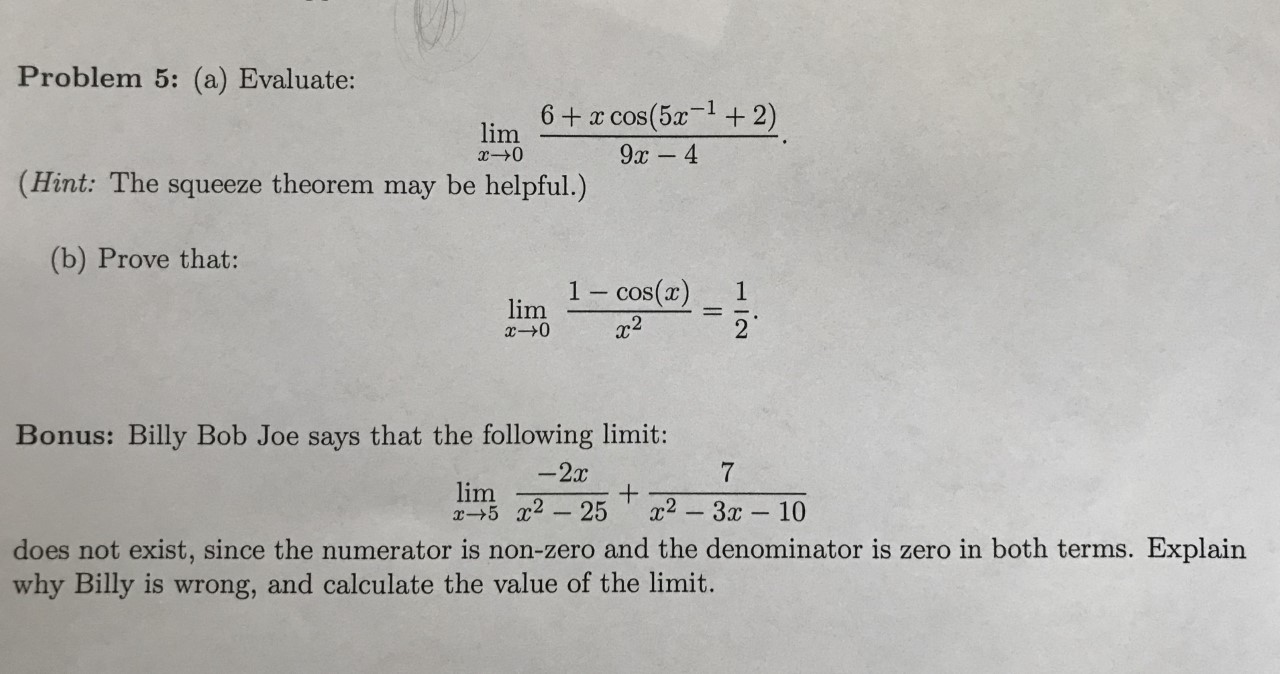# U Problem 5: (a) Evaluate: 6 x cos(5a+ 2) lim x-0 9x- 4 (Hint: The squeeze theorem may be helpful.) (b) Prove that: 1-cos(a) 2 1 lim Bonus: Billy Bob Joe says that the following limit: -2x 7 lim x5 2-25 x2-3x10 does not exist, since the numerator is non-zero and the denominator is zero in both terms. Explain why Billy is wrong, and calculate the value of the limit.

Questionhelp_outlineImage TranscriptioncloseU Problem 5: (a) Evaluate: 6 x cos(5a+ 2) lim x-0 9x- 4 (Hint: The squeeze theorem may be helpful.) (b) Prove that: 1-cos(a) 2 1 lim Bonus: Billy Bob Joe says that the following limit: -2x 7 lim x5 2-25 x2-3x10 does not exist, since the numerator is non-zero and the denominator is zero in both terms. Explain why Billy is wrong, and calculate the value of the limit. fullscreen

### Want to see the step-by-step answer?